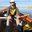Question-and-Answer Resource for the Building Energy Modeling Community
Get started with the Help page

# How to set the minimum distance for collinear points ?In the Engineering Reference from energy plus we can get information about Surface Geometry:

5.4.3 Surface Geometry Shadow calculations first require that the building surfaces be described geometrically. Surfaces are described by the coordinates of their vertices in a three dimensional Cartesian coordinate system. This Right-hand coordinate system has the X-axis pointing east, the Y-axis pointing north, and the Z-axis pointing up (see figure below). The azimuth angle ( ) of a surface is the angle from the north axis to the projection onto the X-Y plane of a normal to the surface (clockwise positive). The surface tilt angle (ϕ) is the angle between the Z-axis and the normal to the surface. The vertices are recorded in counter-clockwise sequence (as the surface is viewed from outside its zone). During surface entry, surfaces are checked for convex or non-convex shape. If non-convex and inappropriate (used as a receiving surface) then a severe error is produced telling the user that shadowing calculations may be inaccurate. Similarly collinear points (or as noted below, points within 1 mm distance) are removed unless removing would make an illegal surface (less than 3 points). But degenerate collinear surfaces should be removed – they make the shadowing routines do extra work which takes extra time. Collinear – points that essentially form a “line” rather than a surface shape. Resolution of 1mm or less – near collinear points. Note that the resolution on surfaces/shadowing is 1 mm – using resolution beyond that will result in truncation of the shadowing.

I have surface with dimension of 2 m x 0.009 m (9 mm). When I run the simulation I got this warning:

* Warning * GetVertices: Distance between two vertices < .01, possibly coincident. for Surface=ZONE_4_SRF_1, in Zone=ZONE_4

* ~~~ * Vertex =(-1.00,-2.00,0.00)

* ~~~ * Vertex =(-1.00,-2.00,9.00E-003)

* ~~~ * Dropping Vertex .

* Warning * GetVertices: Distance between two vertices < .01, possibly coincident. for Surface=ZONE_4_SRF_1, in Zone=ZONE_4

* ~~~ * Vertex =(1.00,-2.00,9.00E-003)

* ~~~ * Vertex =(1.00,-2.00,0.00)

* ~~~ * Cannot Drop Vertex ; Number of Surface Sides at minimum. This surface is now a degenerate surface. . . . So, Is it possible to change the setting of minimum distance to avoid collinear points ?

When I read the reference I thought the minimum surface is 1 mm because it will be collinear points if less than that distance, but it already became collinear points because Distance between two vertices < .01 (10 mm). Or 10 mm is the minimum surface in Energy Plus model?

Thank you very much.

edit retag close merge delete

Sort by » oldest newest most votedThe 0.01 m value is hard coded for collinear points (https://github.com/NREL/EnergyPlus/bl...). There is a difference between the minimum precision (1 mm) with which geometry is modeled and the minimum distance between two vertices. The idea is that the resolution of geometry modeling calculations should be a bit finer than the resolution you can enter for geometry on input. The Engineering reference could be clearer but it is talking about resolution and doesn't actually say what the collinear check is, that is in the warning message.

more

script information:

            // SUBROUTINE INFORMATION:
//       AUTHOR         Linda Lawrie
//       DATE WRITTEN   May 2000


The script was written in 2000 but the information about it still there in engineering reference for 2016/09/30 - EnergyPlus ™ Version 8.6. https://energyplus.net/sites/all/modu...